# STAT 250 Dr Kari Lock Morgan Inference for

• Slides: 34
Download presentation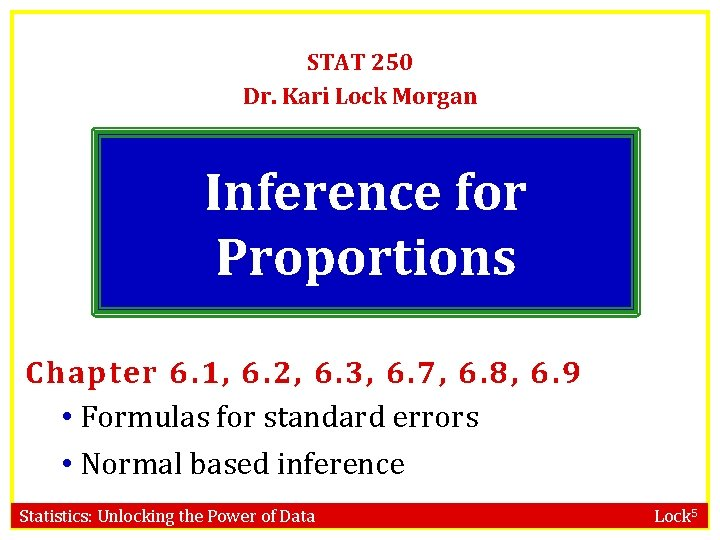STAT 250 Dr. Kari Lock Morgan Inference for Proportions Chapter 6. 1, 6. 2, 6. 3, 6. 7, 6. 8, 6. 9 • Formulas for standard errors • Normal based inference Statistics: Unlocking the Power of Data Lock 5Confidence Interval Formula IF SAMPLE SIZES ARE LARGE… From N(0, 1) From original data Statistics: Unlocking the Power of Data From bootstrap distribution Lock 5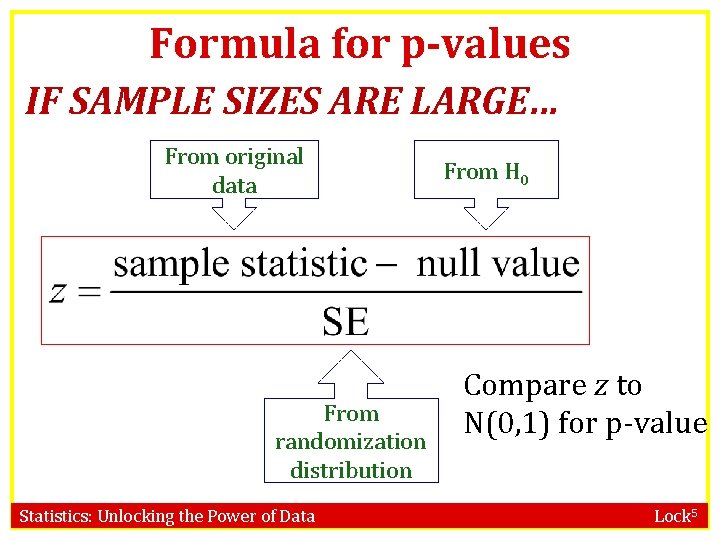Formula for p-values IF SAMPLE SIZES ARE LARGE… From original data From randomization distribution Statistics: Unlocking the Power of Data From H 0 Compare z to N(0, 1) for p-value Lock 5Standard Error • Wouldn’t it be nice if we could compute the standard error without doing thousands of simulations? • We can!!! Statistics: Unlocking the Power of Data Lock 5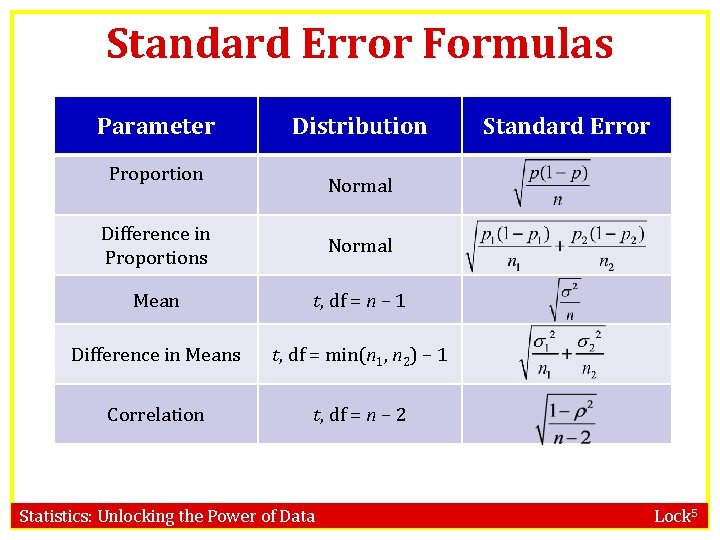Standard Error Formulas Parameter Distribution Proportion Standard Error Normal Difference in Proportions Normal Mean t, df = n – 1 Difference in Means t, df = min(n 1, n 2) – 1 Correlation t, df = n – 2 Statistics: Unlocking the Power of Data Lock 5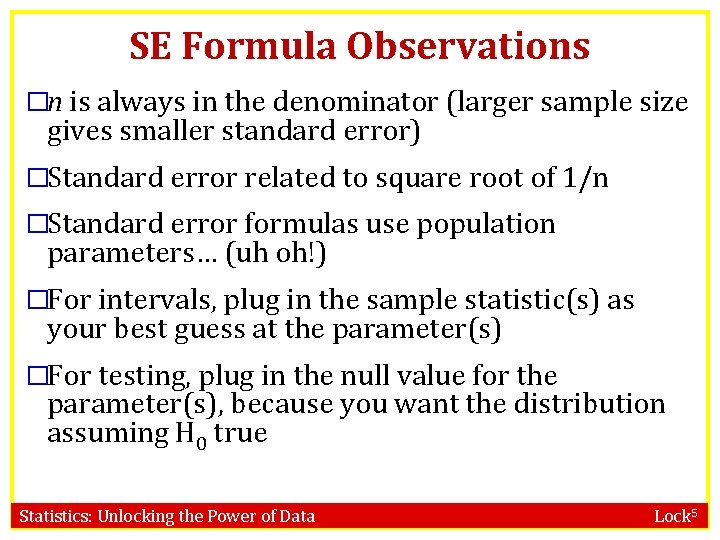SE Formula Observations �n is always in the denominator (larger sample size gives smaller standard error) �Standard error related to square root of 1/n �Standard error formulas use population parameters… (uh oh!) �For intervals, plug in the sample statistic(s) as your best guess at the parameter(s) �For testing, plug in the null value for the parameter(s), because you want the distribution assuming H 0 true Statistics: Unlocking the Power of Data Lock 5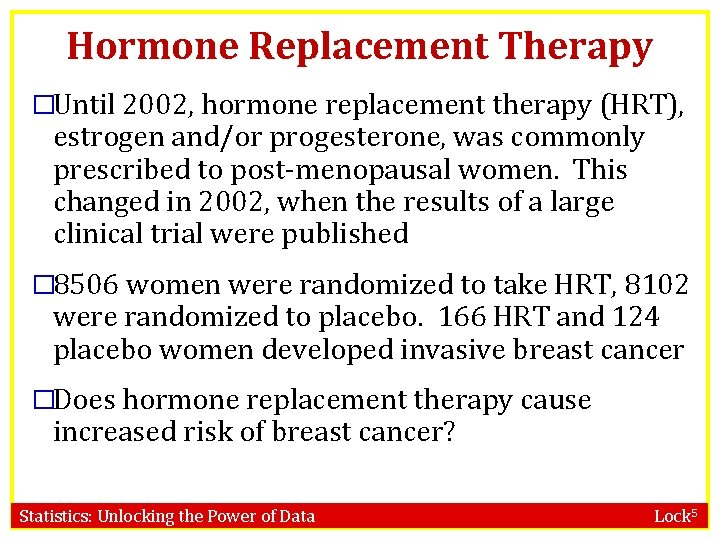Hormone Replacement Therapy �Until 2002, hormone replacement therapy (HRT), estrogen and/or progesterone, was commonly prescribed to post-menopausal women. This changed in 2002, when the results of a large clinical trial were published � 8506 women were randomized to take HRT, 8102 were randomized to placebo. 166 HRT and 124 placebo women developed invasive breast cancer �Does hormone replacement therapy cause increased risk of breast cancer? Statistics: Unlocking the Power of Data Lock 5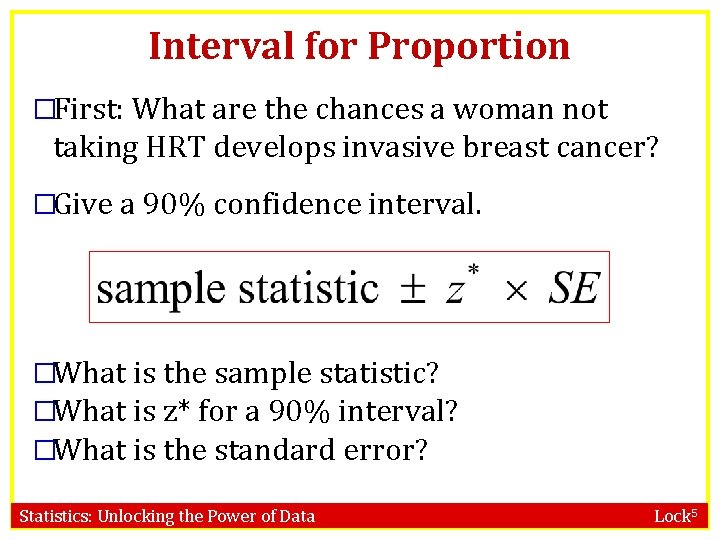Interval for Proportion �First: What are the chances a woman not taking HRT develops invasive breast cancer? �Give a 90% confidence interval. �What is the sample statistic? �What is z* for a 90% interval? �What is the standard error? Statistics: Unlocking the Power of Data Lock 5Sample Statistic � 8506 women were randomized to take HRT, 8102 were randomized to placebo. 166 HRT and 124 placebo women developed invasive breast cancer Statistics: Unlocking the Power of Data Lock 5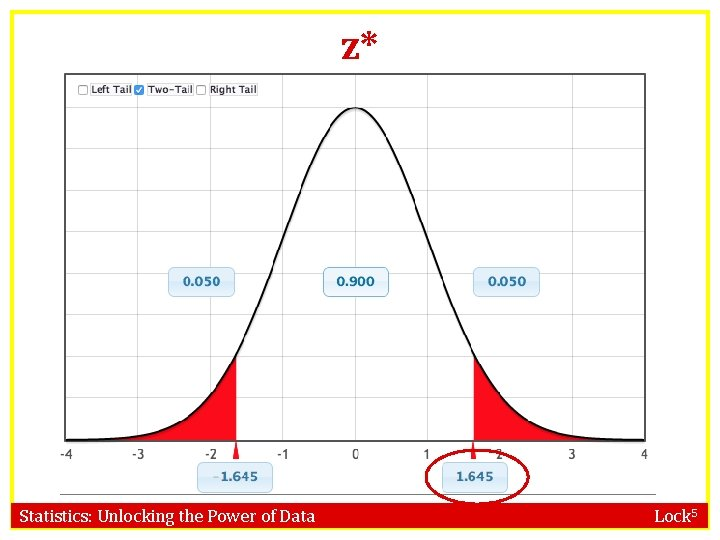z* Statistics: Unlocking the Power of Data Lock 5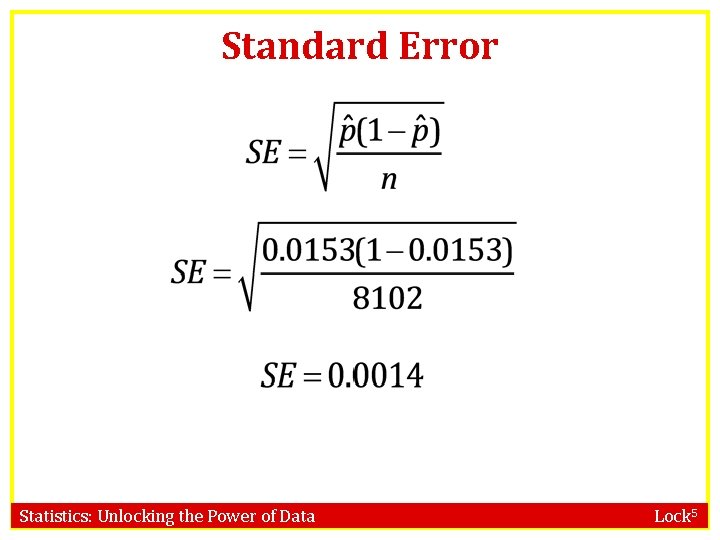Standard Error Statistics: Unlocking the Power of Data Lock 5Standard Error Statistics: Unlocking the Power of Data Lock 5Interval for Proportion Statistics: Unlocking the Power of Data Lock 5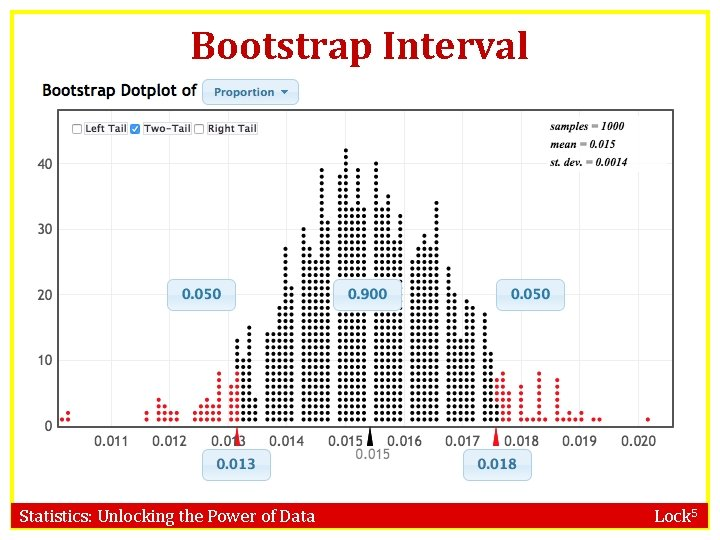Bootstrap Interval Statistics: Unlocking the Power of Data Lock 5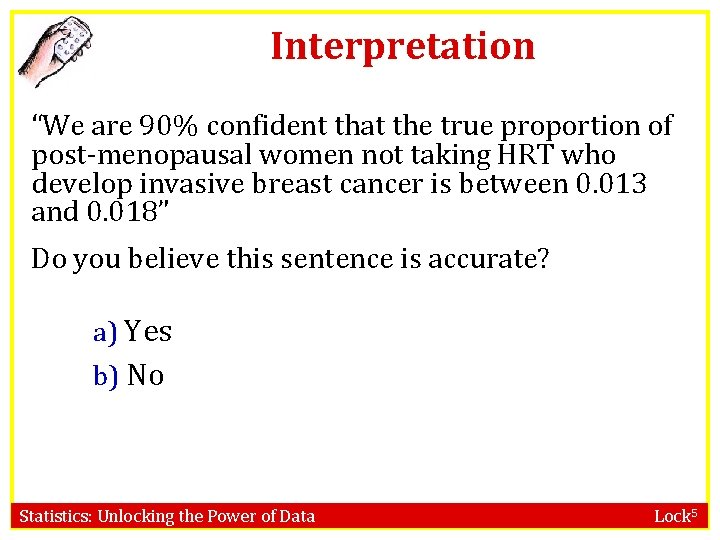Interpretation “We are 90% confident that the true proportion of post-menopausal women not taking HRT who develop invasive breast cancer is between 0. 013 and 0. 018” Do you believe this sentence is accurate? a) Yes b) No Statistics: Unlocking the Power of Data Lock 5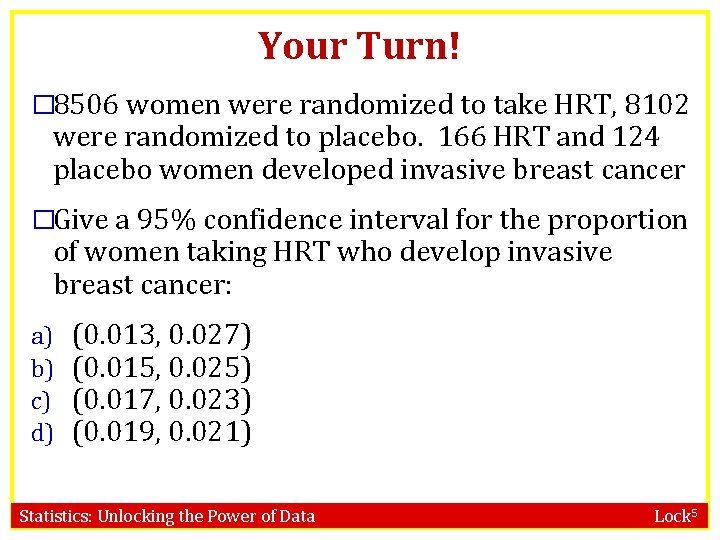Your Turn! � 8506 women were randomized to take HRT, 8102 were randomized to placebo. 166 HRT and 124 placebo women developed invasive breast cancer �Give a 95% confidence interval for the proportion of women taking HRT who develop invasive breast cancer: a) b) c) d) (0. 013, 0. 027) (0. 015, 0. 025) (0. 017, 0. 023) (0. 019, 0. 021) Statistics: Unlocking the Power of Data Lock 5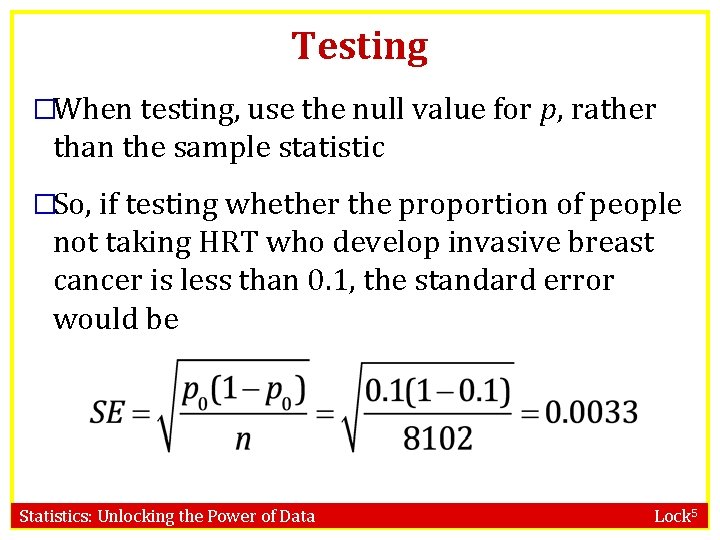Testing �When testing, use the null value for p, rather than the sample statistic �So, if testing whether the proportion of people not taking HRT who develop invasive breast cancer is less than 0. 1, the standard error would be Statistics: Unlocking the Power of Data Lock 5Hormone Replacement Therapy � 8506 women were randomized to take HRT, 8102 were randomized to placebo. 166 HRT and 124 placebo women developed invasive breast cancer �Does hormone replacement therapy cause increased risk of breast cancer? Statistics: Unlocking the Power of Data Lock 5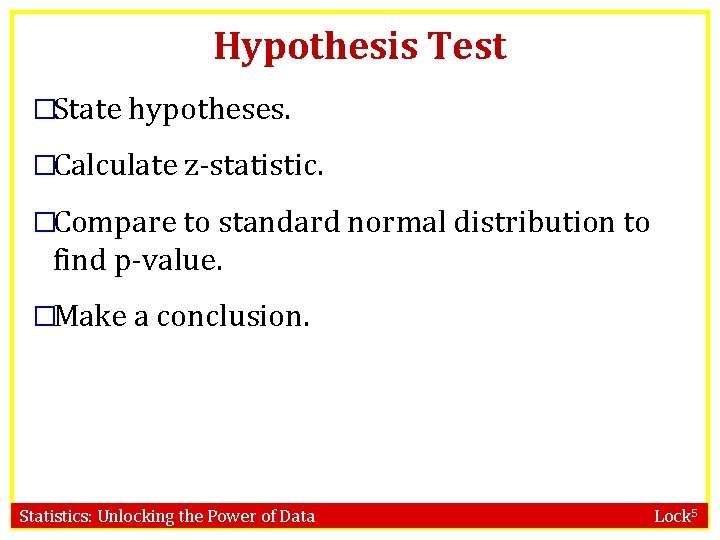Hypothesis Test �State hypotheses. �Calculate z-statistic. �Compare to standard normal distribution to find p-value. �Make a conclusion. Statistics: Unlocking the Power of Data Lock 5Hypotheses Does hormone replacement therapy cause increased risk of invasive breast cancer? p 1 = proportion of women taking HRT who get invasive breast cancer p 2 = proportion of women not taking HRT who get invasive breast cancer a) H 0: p 1= p 2, Ha: p 1≠ p 2 H 0: p 1= p 2, Ha: p 1> p 2 c) H 0: p 1= p 2, Ha: p 1< p 2 b) Statistics: Unlocking the Power of Data Lock 5Calculate z-statistic null value = 0 What to use for p 1 and p 2? ? ? Have to assume null is true… Statistics: Unlocking the Power of Data Lock 5Null Values �Testing a difference in proportions: H 0: p 1 = p 2 �Use the overall sample proportion from both groups (called the pooled proportion) as an estimate for both p 1 and p 2 �Note that this is in between the sample proportions for each group. Statistics: Unlocking the Power of Data Lock 5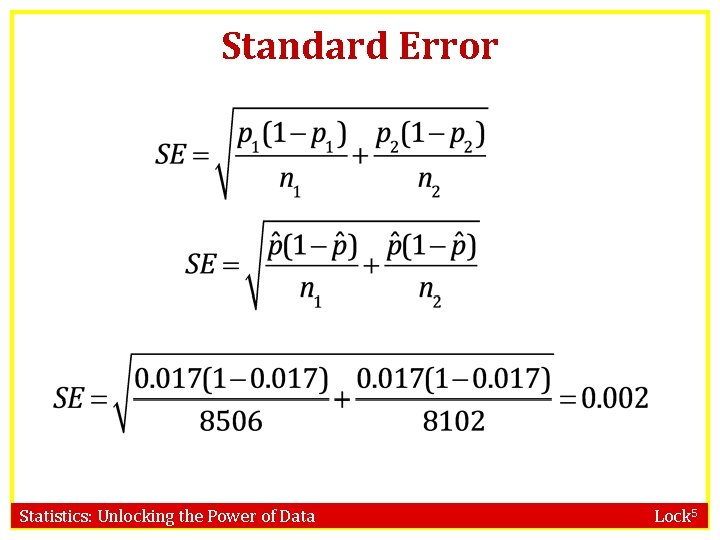Standard Error Statistics: Unlocking the Power of Data Lock 5Standard Error Statistics: Unlocking the Power of Data Lock 5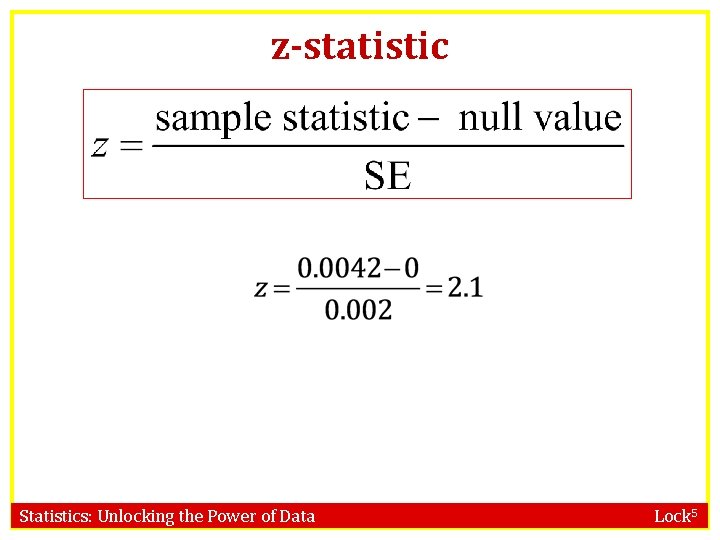z-statistic Statistics: Unlocking the Power of Data Lock 5p-value Statistics: Unlocking the Power of Data Lock 5p-value Statistics: Unlocking the Power of Data Lock 5Conclusion Does this provide evidence that hormone replacement therapy increases risk of invasive breast cancer? a) Yes b) No Statistics: Unlocking the Power of Data Lock 5Your Turn! �Same trial, different variable of interest. � 8506 women were randomized to take HRT, 8102 were randomized to placebo. 502 HRT and 458 placebo women developed any kind of cancer. �Does hormone replacement therapy cause increased risk of cancer in general? a) Yes b) No Statistics: Unlocking the Power of Data Lock 5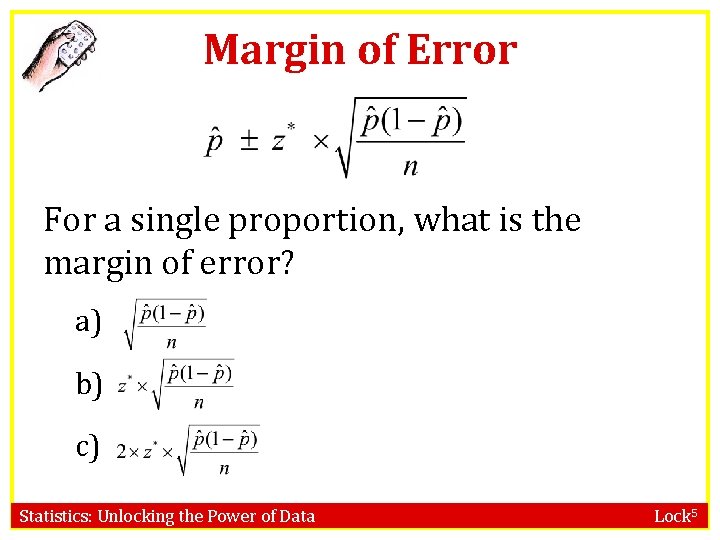Margin of Error For a single proportion, what is the margin of error? a) b) c) Statistics: Unlocking the Power of Data Lock 5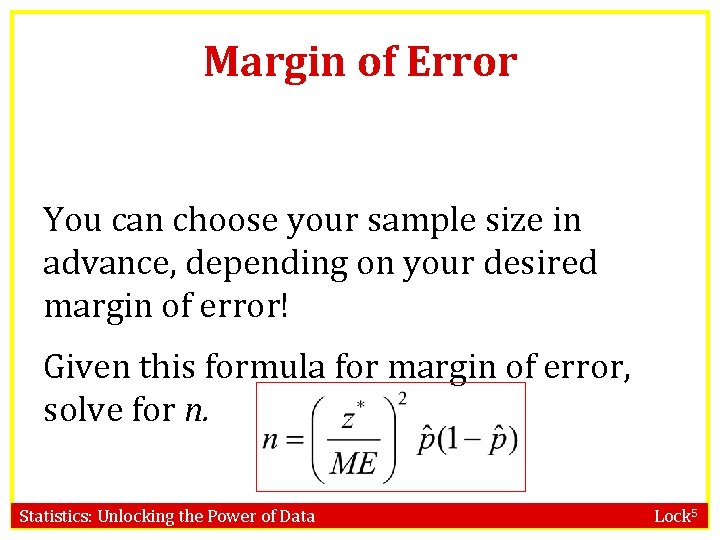Margin of Error You can choose your sample size in advance, depending on your desired margin of error! Given this formula for margin of error, solve for n. Statistics: Unlocking the Power of Data Lock 5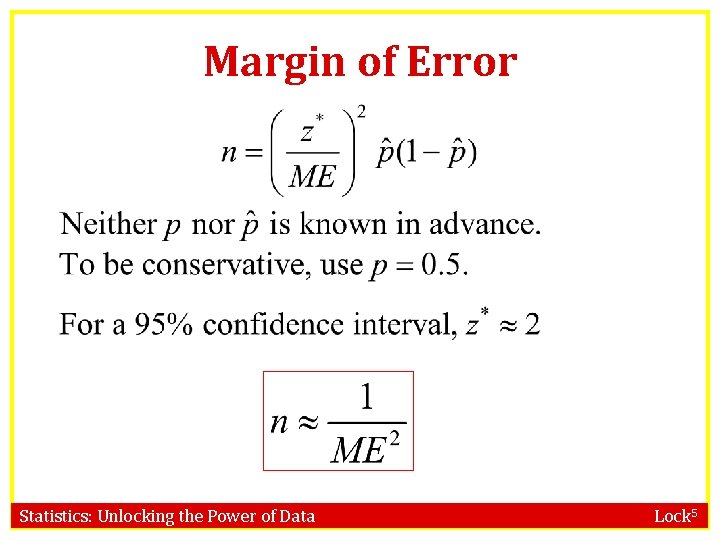Margin of Error Statistics: Unlocking the Power of Data Lock 5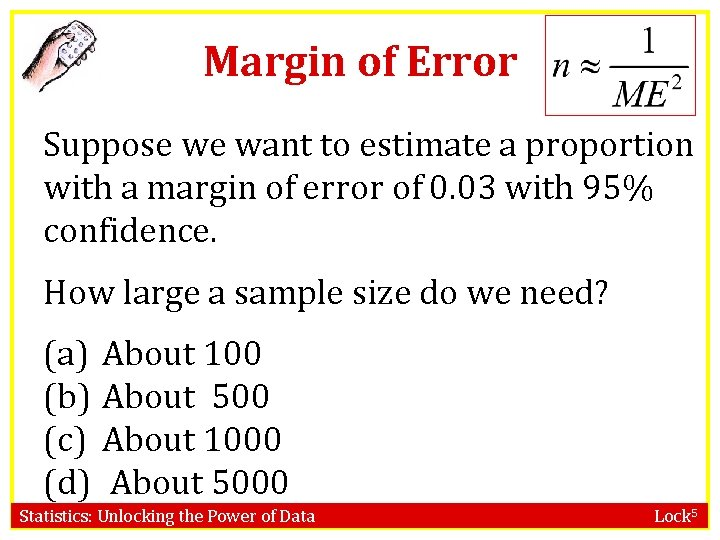Margin of Error Suppose we want to estimate a proportion with a margin of error of 0. 03 with 95% confidence. How large a sample size do we need? (a) About 100 (b) About 500 (c) About 1000 (d) About 5000 Statistics: Unlocking the Power of Data Lock 5To Do �Read Sections 6. 1, 6. 2, 6. 3, 6. 7, 6. 8, 6. 9 �Do HW 6 a (due Friday, 4/10) Statistics: Unlocking the Power of Data Lock 5